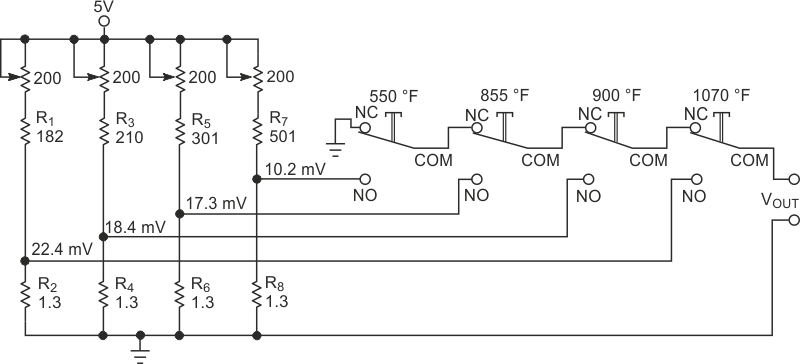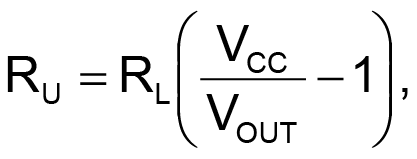# Network imitates thermocouples

Abel Raynus

EDN

Thermocouples find widespread use for temperature measurement in systems. During system design or testing, you must observe the system's response at different temperatures. However, it's inconvenient to heat a thermocouple every time you need to check a system's performance. You can use the simple trick of touching the thermocouple with a hot soldering iron, but this method provides only rough, approximate results. The simple network in Figure 1 allows you to set a number of voltages equal to the thermocouples' outputs at given temperatures. A thermocouple's output is relatively in the tens of millivolts. The low level entails the use of a high-gain amplifier as a signal conditioner. These high-gain amplifiers are sensitive to noise. Susceptibility to noise is not a problem when the amplifier connects to a thermocouple, thanks to the thermocouple's output impedance of approximately 1 Ω. But during system testing, substituting a high-impedance source for the thermocouple can result in noise pickup that can drive the amplifier into saturation. Hence, the output impedance of the thermocouple imitator must be low, and the output must connect to ground between tests.Figure 1. This network allows you to emulate thermocouple outputs at various temperatures.

Figure 1 shows the thermocouple imitator for four temperatures. To obtain low output impedance, you set R2, R4, R6, and R8 to 1.3 Ω. To satisfy the between-tests grounding requirement, the momentary SPDT key switches connect to the chain in a way that, when you press no switch, the output connects to ground. By pressing a switch, you obtain one of the predetermined voltages from dividers R1/R2, R3/R4, R5/R6, or R7/R8 at the output. Assume, for example, imitator-equivalent temperatures of 550, 855, 900, and 1070 °F. You can find the voltages from a Chromel-Alumel thermocouple (Reference 1). But keep in mind that the voltages in the book apply only to a cold-junction temperature of 32°F. The working temperatures are always different, so you must recalculate the voltages. Assuming that the ambient temperature is approximately 100 °F, you can find the thermocouples' output voltages by subtracting 1.52 mV from the 32 °F value (Table 1). You can calculate the values of the divider resistors using the following equation:where RU is the upper divider resistor, RL is the lower divider resistor, VCC is the power-supply voltage, and VOUT is the output voltage. To make the output-voltage adjustment easier, the upper divider resistor consists of a 200 Ω potentiometer in series with a fixed resistor.

Table 1. Correction for cold-junction temperature
 Temperature (°F) Voltage at 320 °F (mV) Voltage at 1000 °F (mV) 550 11.71 10.19 855 18.82 17.3 900 19.89 18.37 1070 23.91 22.39

## Reference

1. The Temperature Handbook, Omega Engineering Inc, 2000.

EDN